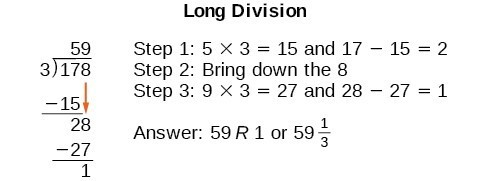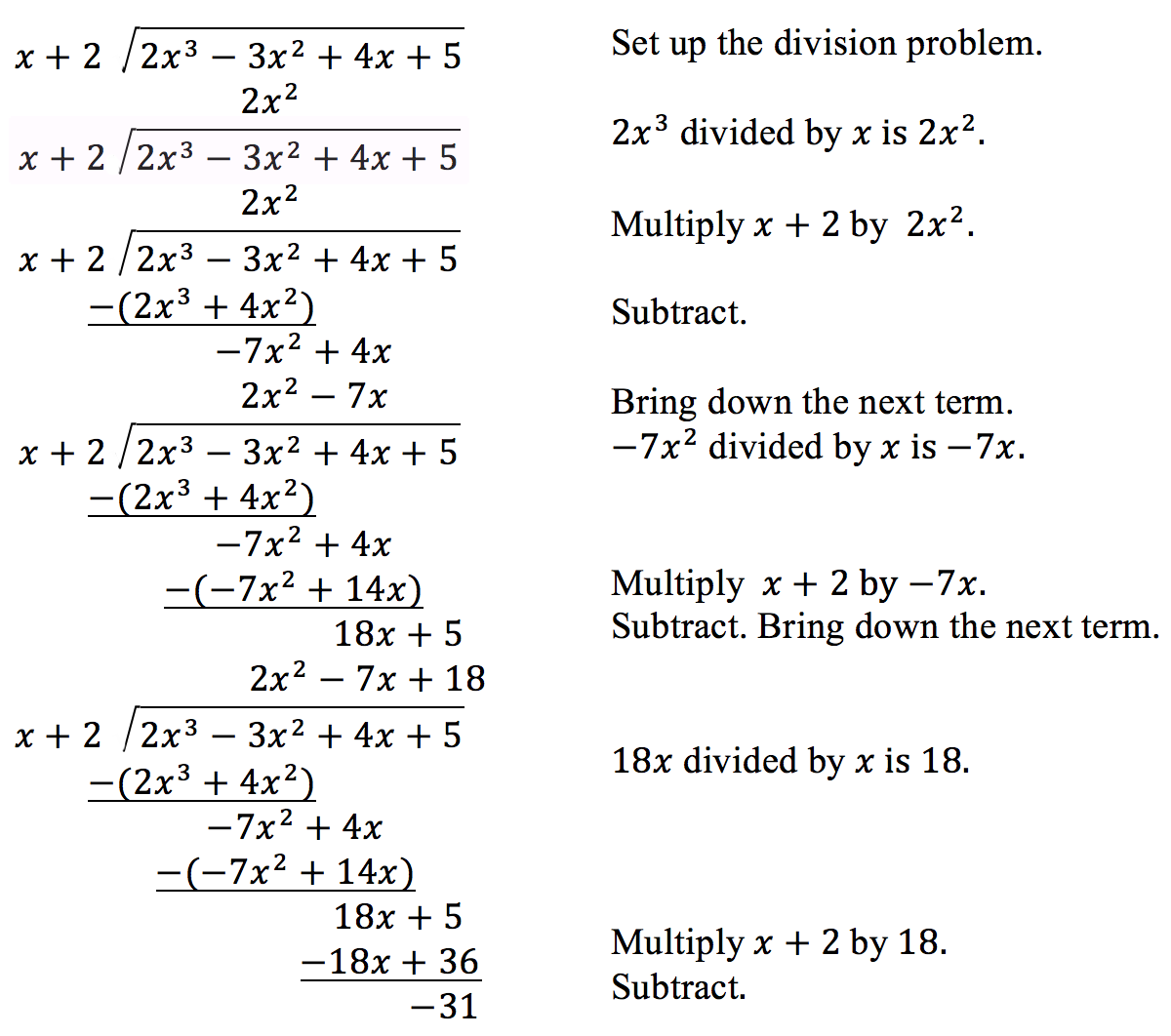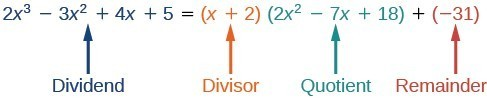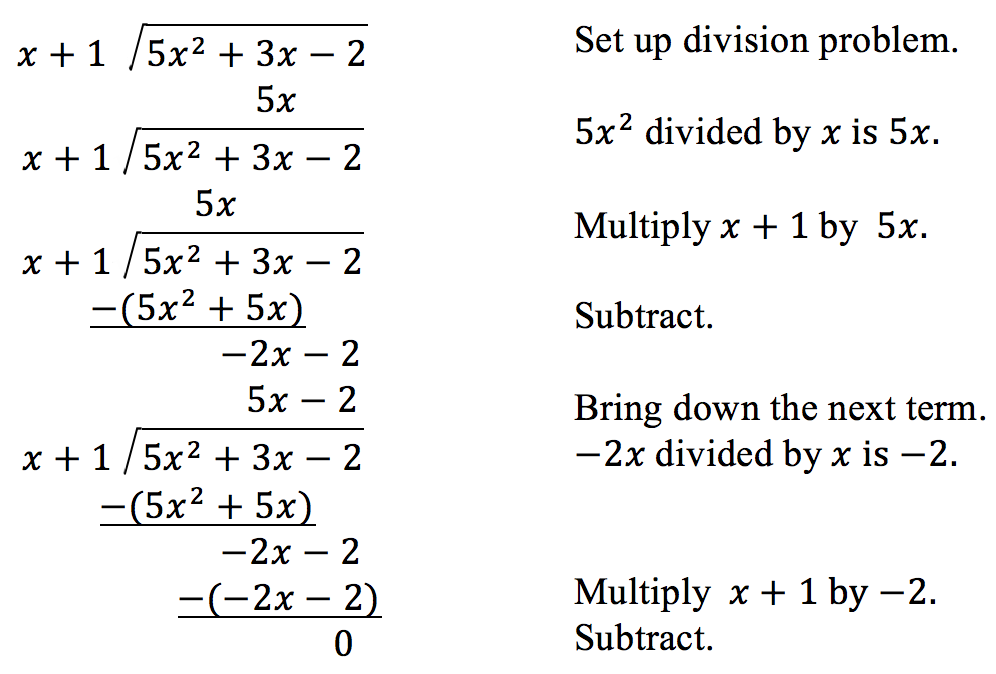## Use long division to divide polynomials

We are familiar with the long division algorithm for ordinary arithmetic. We begin by dividing into the digits of the dividend that have the greatest place value. We divide, multiply, subtract, include the digit in the next place value position, and repeat. For example, let’s divide 178 by 3 using long division.Another way to look at the solution is as a sum of parts. This should look familiar, since it is the same method used to check division in elementary arithmetic.

$\begin{cases}\text{dividend = }\left(\text{divisor }\cdot \text{ quotient}\right)\text{ + remainder}\hfill \\ 178=\left(3\cdot 59\right)+1\hfill \\ =177+1\hfill \\ =178\hfill \end{cases}$

We call this the Division Algorithm and will discuss it more formally after looking at an example.

Division of polynomials that contain more than one term has similarities to long division of whole numbers. We can write a polynomial dividend as the product of the divisor and the quotient added to the remainder. The terms of the polynomial division correspond to the digits (and place values) of the whole number division. This method allows us to divide two polynomials. For example, if we were to divide $2{x}^{3}-3{x}^{2}+4x+5$ by $x+2$ using the long division algorithm, it would look like this:We have found

$\frac{2{x}^{3}-3{x}^{2}+4x+5}{x+2}=2{x}^{2}-7x+18-\frac{31}{x+2}$

or

$2{x}^{3}-3{x}^{2}+4x+5=\left(x+2\right)\left(2{x}^{2}-7x+18\right)-31$

We can identify the dividend, the divisor, the quotient, and the remainder.Writing the result in this manner illustrates the Division Algorithm.

### A General Note: The Division Algorithm

The Division Algorithm states that, given a polynomial dividend $f\left(x\right)$ and a non-zero polynomial divisor $d\left(x\right)$ where the degree of $d\left(x\right)$ is less than or equal to the degree of $f\left(x\right),$ there exist unique polynomials $q\left(x\right)$ and $r\left(x\right)$ such that

$f\left(x\right)=d\left(x\right)q\left(x\right)+r\left(x\right)$

$q\left(x\right)$ is the quotient and $r\left(x\right)$ is the remainder. The remainder is either equal to zero or has degree strictly less than $d\left(x\right).$

If $r\left(x\right)=0,$ then $d\left(x\right)$ divides evenly into $f\left(x\right).$ This means that, in this case, both $d\left(x\right)$ and $q\left(x\right)$ are factors of $f\left(x\right).$

### How To: Given a polynomial and a binomial, use long division to divide the polynomial by the binomial.

1. Set up the division problem.
2. Determine the first term of the quotient by dividing the leading term of the dividend by the leading term of the divisor.
3. Multiply the answer by the divisor and write it below the like terms of the dividend.
4. Subtract the bottom binomial from the top binomial.
5. Bring down the next term of the dividend.
6. Repeat steps 2–5 until reaching the last term of the dividend.
7. If the remainder is non-zero, express as a fraction using the divisor as the denominator.

### Example 1: Using Long Division to Divide a Second-Degree Polynomial

Divide $5{x}^{2}+3x - 2$ by $x+1.$

### SolutionThe quotient is $5x - 2.$ The remainder is 0. We write the result as

$\frac{5{x}^{2}+3x - 2}{x+1}=5x - 2$

or

$5{x}^{2}+3x - 2=\left(x+1\right)\left(5x - 2\right)$

### Analysis of the Solution

This division problem had a remainder of 0. This tells us that the dividend is divided evenly by the divisor, and that the divisor is a factor of the dividend.

### Example 2: Using Long Division to Divide a Third-Degree Polynomial

Divide $6{x}^{3}+11{x}^{2}-31x+15$ by $3x - 2.$

### Solution

There is a remainder of 1. We can express the result as:

$\frac{6{x}^{3}+11{x}^{2}-31x+15}{3x - 2}=2{x}^{2}+5x - 7+\frac{1}{3x - 2}$

### Analysis of the Solution

We can check our work by using the Division Algorithm to rewrite the solution. Then multiply.

$\left(3x - 2\right)\left(2{x}^{2}+5x - 7\right)+1=6{x}^{3}+11{x}^{2}-31x+15$

Notice, as we write our result,

• the dividend is $6{x}^{3}+11{x}^{2}-31x+15$
• the divisor is $3x - 2$
• the quotient is $2{x}^{2}+5x - 7$
• the remainder is 1

### Try It 1

Divide $16{x}^{3}-12{x}^{2}+20x - 3$ by $4x+5.$

Solution